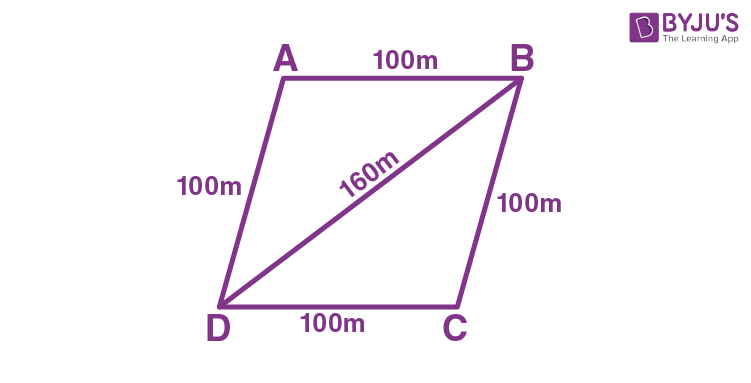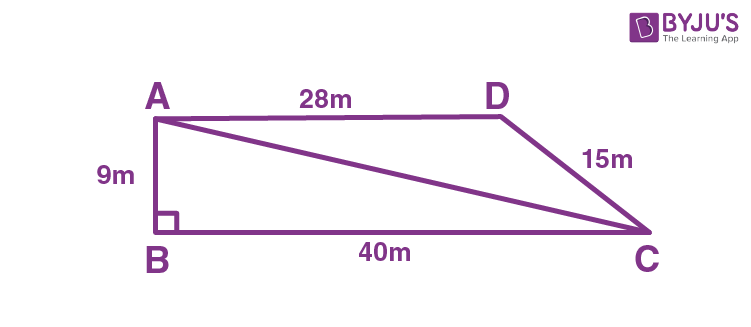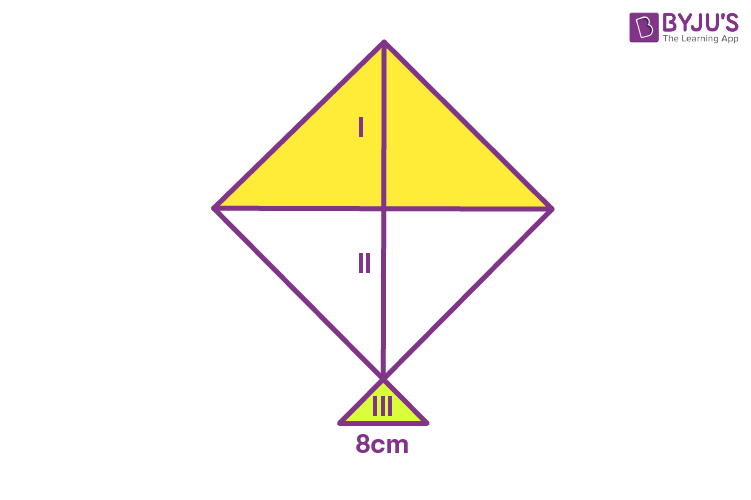# Application of Heron’s Formula in Finding Areas of Quadrilaterals

In geometry, the shapes can be classified into two-dimensional shapes and three-dimensional shapes. 2D shapes are the plane figures, which are made up of two dimensions such as length and breadth, whereas 3D shapes are the solid figures, which are made up of three-dimensions such as length, breadth and height. We know that a triangle is a 2D shape, whose area can be determined by using the formula ½ × b × h, if base and height of the triangle are given. What if only the sides of a triangle are given? How to find the area of a triangle if the side lengths are given?

The solution to this is by applying Heron’s formula. One can find the area of a triangle using Heron’s formula, only if the side measurements are given. Now, let us have a quick recall about Heron’s formula.

## Heron’s Formula – Finding Area of a Triangle

If a, b and c are the sides of a triangle, and s is the semiperimeter of a triangle, then the formula to find the area of triangle using Heron’s formula is:

Area of Triangle = √[s(s-a)(s-b)(s-c)] Square units.

Note: s is the semiperimeter, which means half the perimeter of a triangle, and it is found using the formula:

s = (a+b+c)/2.

## Application of Heron’s Formula in Finding Areas of Quadrilaterals Examples

Heron’s formula is also applicable to find the area of a quadrilateral if the quadrilaterals are divided into triangular parts. Once the quadrilaterals are divided into triangular forms, apply Heron’s formula to find the area of individual triangular parts. After finding the areas of each triangular part, add all the areas to find the area of quadrilateral. Now, let us understand the application of Heron’s formula in finding the areas of quadrilaterals with the help of solved examples.

Example 1:

Masha has a piece of land which is shaped like a rhombus. She wants her one son and one daughter to work on the land to produce different crops. So, she has divided the land into two equal parts. Determine how much area each of them will get for their crop production if the perimeter of the land is 400 m and one of its diagonals is 160 m.

Solution:

Given: ABCD is a rhombus-shaped land.Given that, perimeter = 400 m

Therefore, each side length of rhombus = 400/4 = 100 cm.

Thus, we can take that AD = AB = 100 m

Diagonal, BD = 160 m.

Now, consider the triangle ABD,

The semiperimeter of ∆ ABD, s = (100+ 100+ 160)/2

s = 360/2 m

s = 180 m.

Therefore, the semi perimeter of a triangle = 180m.

Thus, the area of triangle ABD = √[s(s-a)(s-b)(s-c)] square units.

Now, substitute s= 180 m, a= 100, b= 100 and c= 160 in the Heron’s formula, we get

A = √[180 (180 – 100)(180 – 100)(180 – 160)] m2

A = √[180(80)(80)(20)] m2

A = √23040000 m2

A = 4800 m2

Hence, each of them will get land for their crop production of 4800 m2.

Example 2:

The school students staged a rally for a cleanliness campaign. They walked through the lane into two groups. One group walked through the lanes AB, BC and CA, and the other group walked through the lanes AC, CD and DA as shown in the figure. They cleaned the area enclosed within the lanes. Find the total area cleaned by the students (Neglect the width of the lane), if AB = 9m, BC = 40m, CD = 15m, DA = 28m and ∠B = 90º. Also, find which group cleaned more area and how much.Solution:

Given that, AB = 9m, BC = 40m, and ∠B = 90º

Therefore, AC = √(92+402) = √(81+1600)

AC = √1681 = 41m.

Thus, the first group of students cleaned the area of triangle ABC (which is right-angled)

Therefore, the area of triangle = (½)(40)(9) m2

A = 180m2

Hence, the first group of students to clean the lane ABC is 180m2.

Now, the second group of students clean the lane ACD, which is a scalene triangle having side lengths 41m, 28m and 15m.

Now, use Heron’s formula to find the area of ACD.

So, s = (41+28+15)/2

s = 42m

By, using Heron’s formula, A =√[s(s-a)(s-b)(s-c)], we get

A = √[42(42-41)(42-28)(42-15)] m2

A = √[42(1)(14)(27)] m2

A = √15876 m2

A = 126 m2

Hence, the second group of students cleaned the lane ACD is 126m2.

The total area cleaned by all the students = (180+126)m2 = 306m2.

Therefore, the first group of students cleaned more lane areas than the area cleaned by the second group of students.

### Practice Problems

Solve the following problems on the application of Heron’s formula in finding areas of the quadrilateral.

1. A field is in the shape of a rhombus and it has green grass for 18 cows to graze. Determine how much area of grass field each cow will be getting, if each side of the rhombus is 30m and its longest diagonal is 48m.
2. A kite is in a square shape. Its diagonal is 32 cm and its isosceles triangle of base 8 cm and side 6 cm each is made of three different shades as shown in the figure. Find how much paper of each shade has been used in it to make a kite.Stay tuned with BYJU’S – The Learning App and download the app to learn all the Maths-concepts by visualizing more exciting videos.